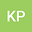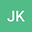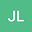Multiple solutions for polyharmonic equations with potential vanishing at infinity
•••Kisoeb Park
Incheon National University
Author ProfileJongrak Lee
Jeju National University
Author Profile## Abstract

We are concerned with the following polyharmonic equation: \begin{equation*} \Delta_p^L u+V(x)|u|^{p-2}u = K(x)f(x,u) and u>0 in \Bbb R^N, \end{equation*} where $1< p<\infty$, $N>Lp$, $L=1,2,\cdots$ and the potential functions $V, K:\Bbb R^{N}\to(0,\infty)$ are continuous. We study the existence and multiplicity of nontrivial positive weak solutions for the problem above via mountain pass theorem and fountain theorem.